# Harnessing deep neural networks to solve inverse problems in quantum dynamics: machine-learned predictions of time-dependent optimal control fields†

Xian Wanga, Anshuman Kumarb, Christian R. Sheltonc and Bryan M. Wong*d
aDepartment of Physics & Astronomy, University of California-Riverside, Riverside, CA 92521, USA
bMaterial Science & Engineering Program, University of California-Riverside, Riverside, CA 92521, USA
cDepartment of Computer Science & Engineering, University of California-Riverside, Riverside, CA 92521, USA
dDepartment of Physics & Astronomy, Material Science & Engineering Program, Department of Chemical & Environmental Engineering, and Department of Chemistry, University of California-Riverside, Riverside, CA 92521, USA. E-mail: bryan.wong@ucr.edu; Web: http://www.bmwong-group.com

Received 10th July 2020 , Accepted 4th September 2020

First published on 8th September 2020

Inverse problems continue to garner immense interest in the physical sciences, particularly in the context of controlling desired phenomena in non-equilibrium systems. In this work, we utilize a series of deep neural networks for predicting time-dependent optimal control fields, E(t), that enable desired electronic transitions in reduced-dimensional quantum dynamical systems. To solve this inverse problem, we investigated two independent machine learning approaches: (1) a feedforward neural network for predicting the frequency and amplitude content of the power spectrum in the frequency domain (i.e., the Fourier transform of E(t)), and (2) a cross-correlation neural network approach for directly predicting E(t) in the time domain. Both of these machine learning methods give complementary approaches for probing the underlying quantum dynamics and also exhibit impressive performance in accurately predicting both the frequency and strength of the optimal control field. We provide detailed architectures and hyperparameters for these deep neural networks as well as performance metrics for each of our machine-learned models. From these results, we show that machine learning, particularly deep neural networks, can be employed as cost-effective statistical approaches for designing electromagnetic fields to enable desired transitions in these quantum dynamical systems.

## I. Introduction

Inverse problems arise in many domains of quantum dynamics, with quantum optimal control being one of the most well-known examples. In the context of molecular systems, the field of quantum optimal control1 seeks to steer a chemical system from a known initial state to a desired target state via an external field, E(t), typically a tailored electromagnetic pulse. Predicting the explicit time-dependence of E(t) is central to providing critical initial conditions for experiments across multiple chemical physics domains including light-harvesting complexes,2–6 quantum information processing,7–9 laser cooling,10,11 and ultracold physics.12,13 As such, the capability to fully harness these optically-driven systems has tremendous potential to grow as we understand how to control the excited-state quantum dynamical processes that govern these systems.

Although several approaches and algorithms have been proposed on optimizing quantum control fields (each with their own purposes and advantages14–17), all of these prior approaches are iterative in nature and require complex numerical methods to solve for these optimal control fields. Due to the nonlinear nature of these dynamical optimization problems, the number of iterations and floating point operations required by these algorithms can be extremely large, leading to extremely slow convergence (even for relatively simple one-dimensional problems16,18). Furthermore, when an optimal control field for a new quantum mechanical system is desired, the entire iteration process has to be re-started de novo since the algorithm has no prior “memory” of previously converged cases. Because of these computational bottlenecks, we wondered whether machine learning, particularly deep neural networks (DNNs), could offer a promising approach for obtaining solutions to this complex, inverse problem in quantum dynamics.

In recent years, machine learning has emerged as a powerful tool in the physical sciences for finding patterns (particularly those that evade human intuition) in high-dimensional data. While the majority of machine learning efforts in the chemical sciences have focused on equilibrium properties such as thermodynamic,19–21 structural,22–25 and ground-state properties26–28 (to name just a select few), considerably less attention has focused on non-equilibrium dynamical processes, such as the explicitly time-dependent optimal fields discussed previously. As such, the use of machine learning in this largely unexplored application of quantum dynamics is a first step towards the design of machine-learned, time-dependent fields for efficiently controlling directed electron/energy transfer in these complex systems.

To this end, we present the first machine learning effort for solving time-dependent quantum control problems in reduced-dimensional chemical systems. These dynamical time-dependent systems pose a unique challenge for conventional machine learning techniques, and we investigate a variety of approaches for predicting optimal control fields, E(t), in these systems. The present paper is organized as follows: Section II briefly outlines the basic concepts of quantum control and the requisite datasets used by the machine learning approaches in our work. Section III describes a neural network approach for predicting the frequency and amplitude content of the power spectrum in the frequency domain (i.e., the Fourier transform of E(t)), whereas Section IV provides a cross-correlation neural network approach for directly predicting E(t) in the time domain. Finally, Section V concludes with a brief discussion and perspective look at potential future applications of our machine learning approach.

## II. Theory and computational methodology

### A. Brief overview of quantum control

Since the main purpose of this work is to harness machine learning techniques for controlling dynamic chemical systems, we only give a brief overview of quantum optimal control and point the interested reader to several topical reviews in this area.29–32 For chemical systems, the quantum optimal control formalism commences with the time-dependent Schrödinger equation for describing the temporal dynamics of nuclei, which in atomic units is given by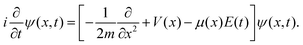(1)
In the equation above, x denotes the reduced coordinate along a chosen reaction path,33–36m is the effective mass associated with the molecular motion along the reaction path,37,38V(x) is the Born–Oppenheimer electronic energy of the molecule, μ(x) is the dipole moment function, E(t) is the time-dependent external electric field, and ψ(x,t) represents the probability amplitude for the motion of the nuclei along the reduced coordinate path. Both V(x) and μ(x) can be obtained from a standard quantum chemistry calculation by carrying out a relaxed potential energy scan.39,40

With x and V(x) properly chosen/computed, eqn (1) allows us to mathematically answer the question: “given an electric field E(t), how does an initial state, ψ0(x,t = 0), evolve after some final time T has elapsed?” However, as mentioned in the Introduction, the field of quantum optimal control is an inverse problem and instead seeks the answer to the “inverse” question: “if we want to reach a desired final state ψN−1(x,t = T) at time T (after N − 1 propagation steps), what does the functional form of E(t) look like?” To be more mathematically precise, quantum control seeks the functional form of an external electric field, E(t), that maximizes the functional J[ψN−1,E] given by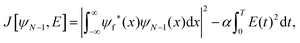(2)
where ψf is a known desired final target wavefunction (given by the user), and ψN−1 is obtained after applying N − 1 successive propagation steps of the time-dependent Schrödinger equation (i.e., eqn (1)). It should be noted that the first term in eqn (2) is essentially a measure of the similarity of the final target and the propagated wavefunction. The second term in eqn (2) is a fluence and acts as a penalty to prevent unphysically large values of the electric field, where α is a positive constant (set to 0.001 in this work) to be chosen by the user. Providing accurate and efficient answers to this inverse question is the ultimate goal of the machine learning approaches described in this work.

### B. Generation of datasets used for machine learning

To generate the data required for our machine learning approaches, we utilized the NIC-CAGE (Novel Implementation of Constrained Calculations for Automated Generation of Excitations) program developed in our previous work.41 Given a potential, V(x), this program iteratively calculates a numerical representation of E(t) that enables a ≈100% transition probability between two desired electronic transitions (which, in this work, are the ground and first-excited state, schematically shown in Fig. 1a and b). In simple terms, our NIC-CAGE program can be seen as a black box that accepts potential functions, V(x), as input and subsequently outputs optimal electric fields, E(t), corresponding to the inputted potentials. It is important to note that the optimal electric field, E(t), can also be represented in the frequency domain as a power spectrum, σ(ω), by applying a fast Fourier transform (FFT) to E(t) (cf.Fig. 1c). In this work, we seamlessly switch between the time and frequency domains to provide different machine learning approaches for predicting optimal control fields in these dynamic systems.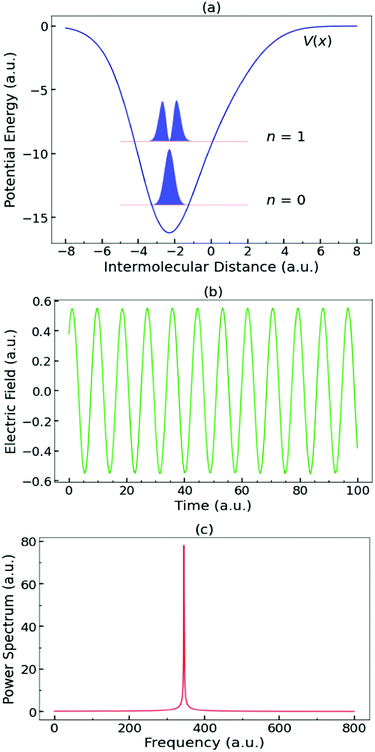Fig. 1 Schematic example of (a) a potential well, V(x), as a function of intermolecular distance x. The horizontal dashed lines denote the energy levels of the ground and first excited state, and their respective probability wavefunctions, |ψ(x)|2, are depicted as blue curves above the energy levels; (b) the optimal electric field E(t) required to excite the transition between the ground and the first excited state; (c) the corresponding power spectrum σ(ω) as a function of frequency ω, obtained from the fast Fourier transform of E(t).

While the NIC-CAGE program41 can obtain transition probabilities with notable accuracy (typically over 97%), it can take hundreds of iterations (or longer) to converge to the final electric field for each potential. Moreover, as mentioned in the Introduction, when a new potential is inputted, the iteration process has to be re-started anew since the program has no prior memory of previously converged cases. For these reasons, the prediction of optimal electric fields for a general potential energy function is a natural application for a data-driven solution. To generate a large dataset for our machine learning approaches, a vast number of potentials were generated as input to the NIC-CAGE program to produce corresponding optimized electric fields. These potential-field pairs served as the training, validation, and test sets for our DNNs.

Our complete dataset consisted of 36118 randomly generated potential functions, V(x), each of which was evaluated across 192 points in one dimension. For all of these potential functions, the effective mass, m, and dipole moment, μ(x), were set to 1 and x, respectively. To enable statistical flexibility in this dataset, each potential was constructed by the summation of three Gaussian functions with varying amplitudes, widths, and centers, according to the following equation:42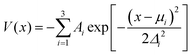(3)
Specifically, our dataset was created by randomly sampling each of the parameters with the following ranges: amplitude A ∈ [1, 10], center μ ∈ [−3, 3], and width Δ ∈ [0.5, 2]. As such, each potential function can be fully described by nine randomly generated parameters. In addition, we also visualized this parameter space and found that all parameters were evenly distributed within the selected range, indicating that the randomly generated potential functions sufficiently span this phase space (cf.Fig. 2). Each of the 36118 potential functions was inputted into the NIC-CAGE code, which resulted in an optimized electric field evaluated across 30000 points in the time domain.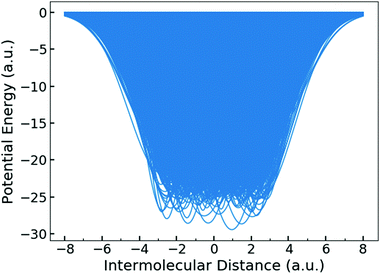Fig. 2 Plot of all 36118 potentials sampled in this work. The center region of the V(x) space is densely packed and fully sampled, indicating that the full set of these potentials sufficiently explores this phase space. The side regions of the figure are not filled by the potential energy curves since the range of the Gaussian centers, μ, were intentionally kept small to prevent the wavefunctions from spreading outside the x ∈ [−8.0, 8.0] range.

Of the 36118 potentials examined in this work, 26000 were used for the training set, 5000 were utilized for the validation set, and the remaining 5118 potentials were designated for the test set. We ensured that the number of potentials used in the training, validation, and test sets were exactly the same for each training instance to ensure that the results could be compared.

### C. General neural network architectures

We employed feedforward neural networks (FNNs) for this work due to their simplicity as well as their ability to learn complicated mappings between input and target spaces. The FNNs used here can be classified as deep network architectures, with the depth in each network arising from the stacking of multiple hidden layers. Each hidden layer accepts output from the previous layer as input, and returns a non-linear activation as the output. It is worth noting that the predictive accuracy of the FNNs can be sensitive to several key hyperparameters and training methods, such as the number of hidden layers, the number of nodes in each layer, the learning rate, and the regularization method. As such, multiple models and parameters were tested in this work (i.e., we also tested convolutional neural networks but found that the best results were obtained with FNNs), and we only present FNN architectures and parameters in Sections III and IV with the best performance.

## III. Neural networks for predicting the resonance frequency and amplitude, σ(ω)

In this section, we describe our first machine learning approach, which utilizes FNNs to predict the frequency and amplitude of the power spectrum, σ(ω), in the frequency domain. As briefly mentioned in Section IIB, the power spectrum is obtained by a standard numerical procedure in which a fast Fourier transform of a properly converged E(t) is first computed, followed by taking its absolute value. It is worth mentioning that because of the last absolute value operation, the phase of the original electric field is inherently lost and, therefore, only the amplitude and frequency were predicted with our FNNs in this section. To this end, we utilized two independent FNNs to separately learn the frequency and amplitude, and a schematic of the FNN architecture used for both of these predictions is shown in Fig. 3.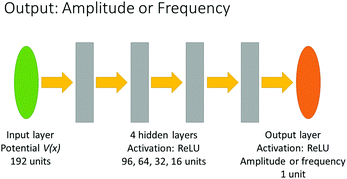Fig. 3 Architecture of the FNN used to predict the amplitude and resonance frequency of the power spectrum, σ(ω). The FNN starts with an input layer composed of 192 units (which correspond to the potential, V(x), evaluated across 192 points), followed by four hidden layers of various sizes. The output layer is composed of 1 unit to predict either the amplitude or resonance frequency of σ(ω).

Upon closer inspection of the original test set used in this work, we noticed that 66 of the optimal E(t) fields had extremely large amplitudes (i.e., these specific electric fields were characterized by amplitudes that were an order of magnitude larger than the average E(t) in the test set). Since electric fields with these large amplitudes are difficult to construct in a realistic experiment, we eliminated these 66 data points (they account for only 1.29% of the 5118 data points), and we designated this dataset as our pruned test set. The input for each of our independent FNNs was the potential V(x) (consisting of 192 data points), whereas the output was the single value of the frequency or amplitude, as depicted in the last step of Fig. 3. Both of these two FNNs were constructed and trained using a Tensorflow43 backend with GPU acceleration powered by NVIDIA CUDA libraries.44 In each FNN model, all of the weight matrices were initialized with random values satisfying a normal distribution, while all the biases were initialized to 0.001. We chose our loss function based on the definition of the mean square error (MSE), given by the following equation: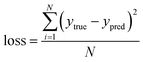(4)
where N is the mini-batch size, ytrue is the true frequency/amplitude of σ(ω) obtained from the NIC-CAGE program, and ypred is the frequency/amplitude predicted by the machine learning algorithm. An L2 regularization of the weights was applied to prevent overfitting, and the built-in Adam optimizer was utilized. The training, validation, and test sets were kept the same size, and after several tests, we found that the optimal learning rates and regularization coefficients were different for these two FNNs, while all other optimal hyperparameters had the same values. Table 1 summarizes the optimal hyperparameters used in each of these FNNs.

Table 1 Hyperparameters and settings of the FNNs used for predicting the amplitude and frequency of the optimized E(t)
Output purpose Amplitude Frequency
Neural network structure Feedforward Feedforward
Activation ReLU ReLU
Learning rate 0.0001 0.0005
Loss function MSE MSE
Regularization L2 L2
Regularization coefficient 0.0001 0.0005
Mini-batch size 1024 1024
Number of hidden layers 4 4
Number of units in hidden layers 96, 64, 32, 16 96, 64, 32, 16

Fig. 4 depicts the results of our machine-learned amplitudes and frequencies. The diagonal line in each plot represents a perfect match between the machine-learned predictions and true values (obtained with 1000000 epochs). To further quantify this performance, we computed a coefficient of determination (R2) for measuring the similarity between ypred and ytrue: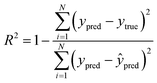(5)
where N is the batch size, and ŷpred is the average of all the ypred values in the batch. A perfect agreement between ypred and ytrue yields an R2 value of 1. As visually shown in Fig. 4 and from the R2 values listed in Table 2, our machine learning approaches were more accurate in predicting the resonance frequency compared to the amplitude. This difference in performance suggests that the machine-learned mapping from the potential to the amplitude is much more complicated than the mapping from the same potential to the resonance frequency. More concretely, the frequency has a more clear/intuitive physical meaning, which is equal to the energy difference between the ground- and first-excited state. However, the amplitude is much more sensitive to the underlying shape of the potential, V(x), and this sensitivity contributes to the error in predicting the amplitude with our neural network. This difference in predictive performance can also be seen by comparing the figures of the R2 values vs. the epoch number on the validation set. In particular, the R2 values for predicting the frequency show a smooth progression, while that for the amplitude fluctuates significantly as shown in Fig. 5c and d. We also investigated the sensitivity of our results to the size of our training set and found that the accuracy of the machine-learned predictions decreased with the training set size. Specifically, when the training set was reduced to only 10000 potentials, the R2 values for predicting the resonance frequency and amplitude in the same validation set decreased to 0.93 and 0.41, respectively. As such, these statistics showed that a sufficiently large training set was necessary to enable accurate machine-learned predictions for these optimal control fields.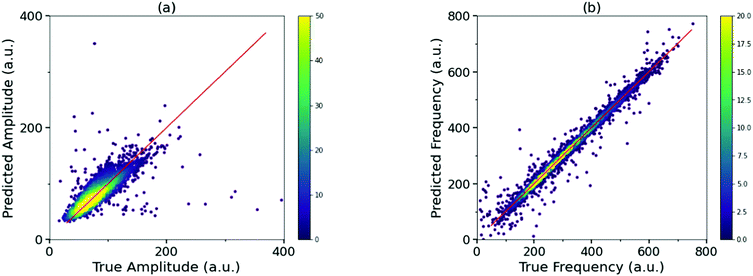Fig. 4 Scatter density plots of the machine-learned predicted vs. true (a) amplitudes and (b) frequencies. The diagonal line in each plot represents a perfect match between the machine-learned predictions and true values. Both plots were obtained with 1000000 epochs. The vertical color bar in each sub-plot indicates the density of the data points.
Table 2 FNN metrics for predicting the amplitude and frequency, respectively
Output Amplitude Frequency
Number of epochs for best performance ∼1000000 ∼1000000
Loss on original test set 509.1925 286.1925
R 2 for pruned test set 0.6036 0.9814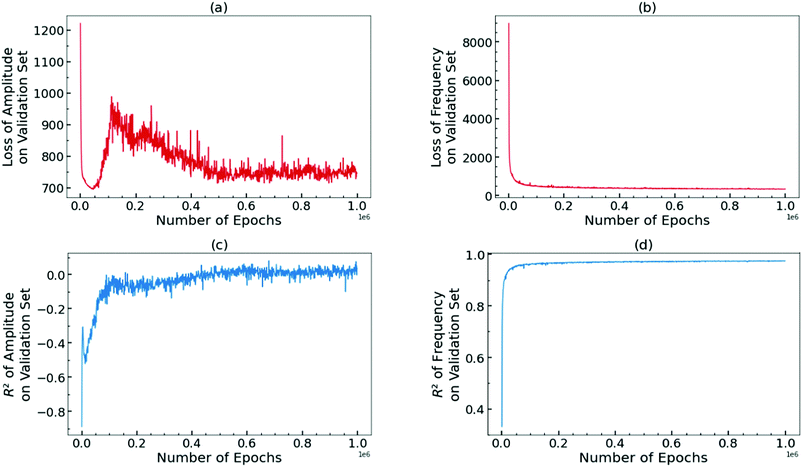Fig. 5 Plot of loss vs. number of epochs for FNN predictions of (a) the amplitude and (b) resonance frequency. R2 values for the FNN-predicted (c) amplitude and (d) resonance frequency. All plots were generated from the validation dataset.

We also explored the option of predicting the entire power spectrum instead of just the primary resonance frequency and amplitude. Several attempts were made along those lines, including reducing the size of the output to 800 rather than 15000 (since the resonance peaks typically had small frequencies), choosing a cross-entropy loss function instead of the MSE, fixing the lineshape of the output to be a Gaussian or symmetric Lorentzian to reduce the number of units (i.e., to 3) required in the output layer to predict the power spectrum, etc. Unfortunately, all of these attempts failed in predicting the correct amplitude of the power spectrum, although some of them were quite successful in predicting the resonance frequency. We attribute these failures to the sharpness of the resonance peak in the power spectrum. Due to the limited resolution inherent to the discrete σ(ω) data, each peak only consisted of a few data points and, therefore, the linewidth was not well-resolved. In other words, since the linewidth of the resonance peak in σ(ω) was inherently imprecise, the FNN was unable to converge to a proper mapping of the power spectrum. In addition, we also tested one-dimensional convolutional neural networks (CNNs) for predicting the frequency and the amplitude as well as the entire power spectrum. Unfortunately, the results obtained with CNNs were less accurate than those obtained with the FNN approaches used here. Because of these limitations, we investigated other FNN architectures to learn mappings between V(x) and E(t) in the time domain. This is motivated by the fact that if E(t) can be accurately predicted using FNNs in the time domain, σ(ω) could also be accurately resolved (since σ(ω) is merely the Fourier transform of E(t)), and we discuss these strategies in the next section.

## IV. Neural networks for directly predicting the electric field, E(t)

While Section III focused on predicting the power spectrum, σ(ω), in the frequency domain, we now investigate whether the electric field in the time domain, E(t), can be predicted with a machine learning approach. Predicting these dynamic fields as an explicit function of time presents unique challenges for machine learning approaches. In particular, while σ(ω) in the frequency domain contains no phase information, E(t) in the time domain does contain an explicit phase dependence (cf.Fig. 1b) that requires additional care, which we discuss in further detail below.

To predict E(t) as an explicit function of time, we constructed an FNN with three hidden layers, which was trained with the same GPU-accelerated Tensorflow43 backend and NVIDIA CUDA libraries44 used in Section III. Our FNN, depicted in Fig. 6, was designed such that the number of units increases as data flows towards the output layer. Specifically, the input layer was composed of 192 units (which correspond to the potential, V(x), evaluated across 192 points), followed by three hidden layers having 300, 500, and 750 units, respectively. The output layer, which outputs the electric field as a function of time, was composed of 1000 (or fewer) units. Similar to the FNN used in Section III, the activation for both the input and hidden layers was chosen to be a ReLU function without any leaky or bounded modification. Since the output array is expected to be sinusoidal with a zero base, the activation of the output layer was chosen to be a tanh function to enable the output of negative values. All of the weight matrices were initialized with random values satisfying a normal distribution, while all the biases were initialized to 0.001. We chose the same loss function (cf.eqn (4)), L2 regularization, and Adam optimizer described previously in Section III for our FNN. Based on several tests of our data, we found that a regularization coefficient 0.001 was optimal for balancing regression speed and overfitting.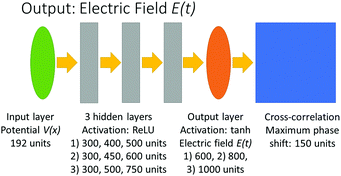Fig. 6 Architecture of the FNN used to predict the electric field, E(t). The FNN starts with an input layer composed of 192 units (which correspond to the potential, V(x), evaluated across 192 points), followed by three hidden layers of various sizes. The output layer is composed of 1000 (or fewer) units and is directly interfaced with a cross-correlation algorithm to predict the final electric field, E(t).

For the specific case of excitations from the ground to the first-excited state, we noticed that the optimal electric field, E(t), could be closely approximated with a sinusoidal function (with a single frequency and amplitude) regardless of the potential function used. Because of this periodicity, the time-dependent trends in these electric fields could be accurately captured by only considering a smaller portion of the entire periodic signal. To this end, we only extracted 1000 (or fewer) representative data points within the entire 30000-point electric field for our output set. This simplification allowed us to train our machine learning models more easily due to constraints in holding this large amount of data in RAM, the immense computing time, and associated GPU resources.

In the same spirit of reducing the number of physically relevant parameters needed for our machine learning efforts, we also explored whether the transition probability was sensitive to the specific phase factors or amplitudes directly obtained from the NIC-CAGE code. To test the first assumption, we inputted several electric fields with different phase shifts, φ (but having the same optimized frequency and amplitude that gives the desired transition), as an initial guess into the NIC-CAGE code (cf.Fig. 7a). All of these phase-shifted electric fields gave a transition probability close to unity (with the NIC-CAGE code exiting immediately without further iterations), indicating that the transition probability was not dependent on the phase. However, when we tested the second assumption by inputting electric fields with different amplitudes as an initial guess into the NIC-CAGE code (cf.Fig. 7b), we observed a completely different phenomenon. Specifically, all of these initial conditions resulted in several subsequent iterations that eventually reverted/converged to the same optimal E(t) form (cf.Fig. 7c). Taken together, both of these benchmark tests indicate that the optimal E(t) is insensitive to the phase but highly dependent on the amplitude. As such, these tests allow us to construct a streamlined FNN using a cross-correlation technique for predicting E(t) in the time domain (without having to directly predict the phase factor, since it has no physical effect on the dynamics), which we describe in further detail below.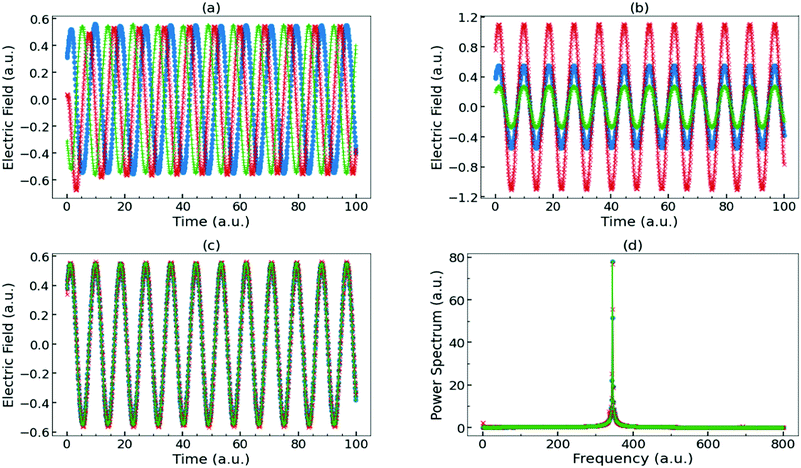Fig. 7 (a) Optimized electric field, E(t), with various phase shifts, φ. The blue data points denote the optimized E(t) obtained directly from the NIC-CAGE code. The red curve is the same E(t) with a phase shift of π/2, and the green curve is E(t) with a phase shift of π. When each of these electric fields is used as initial guesses for propagating the time-dependent Schrödinger equation, all of them gave a transition probability close to unity (which shows that the transition probability is insensitive to the phase, φ). (b) Optimized electric field, E(t), with various amplitudes. The blue data points denote the optimized E(t) obtained from the NIC-CAGE code, and the red and green curves denote the same E(t) with amplitudes multiplied by 2 and 0.5, respectively. When each of these electric fields was used as initial guesses for time propagation, all of them reverted/converged back to the E(t) with the original amplitude shown in panel (c), which indicates that the transition probability depends critically on the electric field amplitude. (d) Power spectra, σ(ω), of the various E(t) fields depicted in (a), showing that they coincide with each other, as expected.

For the ground to first-excited state transitions examined in this work, each of the optimal control fields, E(t), can be nearly characterized by a single amplitude, frequency, and phase, φ. Since we showed previously that the transition probability is insensitive to φ, a conventional neural network may be unable to learn any patterns that map between V(x) and φ, since the phase is arbitrary and has no physical meaning. To sidestep this difficulty, we used a cross-correlation approach to shift the predicted E(t) by a series of different phase values. In essence, this generates multiple E(t) functions with exactly the same frequency and amplitude but with a variety of different phases. To this end, 150 shift-matrices were constructed by shifting the identity matrix along rows with a “roll” function. To more concretely illustrate how we automated these phase shift operations in our machine learning approach, we denote E(t) as a row vector given by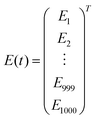(6)
Therefore, E(t) can be trivially written as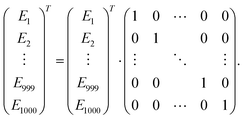(7)

By shifting the diagonal entry of the identity matrix, the phase of E(t) can be “rolled” or shifted as follows: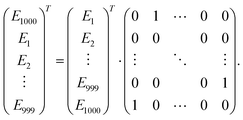(8)
Using this approach, the predicted E(t) can be shifted along the time axis by 0 to 150 increments when multiplied by the matrix in eqn (8). As such, each output array was spanned to a set of 150 arrays with exactly the same frequency and amplitude, but with different phases, φ. We also tested the accuracy of this approach by using a smaller number of shift matrices but found that at least 100 of these arrays were needed to sufficiently sample the entire phase space of φ ∈ [0, 2π] (i.e., each new array shifts the phase, φ, by at least 2π/100, and 100 or more arrays were necessary to ensure that the phase within the interval [0, 2π] was sufficiently represented to give accurate results). With these 150 shift matrices in hand, the MSE loss was computed for each prediction, and when the phase of the prediction matched that of the true E(t), the MSE loss was minimized. The weights and biases of the neural network were then updated using a back-propagation algorithm based on the minimum loss value. It is worth noting that our cross-correlation approach was only used to train the neural network, and after the neural network was successfully trained, the cross-correlation procedure was no longer needed to process/predict new data.

We optimized some of the hyperparameters used by our cross-correlation neural network approach for the training set, and the optimal learning rate was chosen to be 0.0001. A mini-batch of 1024 input arrays was chosen from the training set for each training epoch, and the training set was fully shuffled after each epoch. Since the electric fields outputted by the NIC-CAGE program had amplitudes on the order of ∼0.01, all of the electric fields were multiplied by 80 to avoid numeric underflows and allow the weights and biases to converge faster in our machine learning algorithms. We chose a scaling factor of 80 to ensure that the processed electric field would not exceed 1, since the tanh function used in our output layer has a range of [−1, 1]. Table 3 summarizes our selection of hyperparameters and settings used to predict E(t) in the time domain.

Table 3 Hyperparameters and settings of the FNN used for predicting E(t) in the time domain
Neural network structure Feedforward
Activation ReLU (hidden layers)
tanh (output layer)
Learning rate 0.0001
Loss function MSE
Regularization L2
Regularization coefficient 0.001
Mini-batch size 1024
Multiplicative pre-factor of E(t) 80
Maximum phase-shift in cross-correlation 150 increments

To reduce the large RAM requirements and computational effort for our machine learning algorithms, we reduced the number of units for predicting E(t) to 600 and 800 from our original 1000-output-layer-unit model. The number of hidden layer units were also reduced to 300, 400, 500, and 300, 450, 600, while the size of the input layer remained the same. This reduction in the number of points had a negligible effect on predicting the frequency of E(t), as shown in Fig. 8a–c. In these plots, the predicted E(t) is shifted with the proper phase to allow a more straightforward comparison. Both the frequency and amplitude agree well, and these results show that our cross-correlation approach is able to address the previous issues associated with the random phase of E(t). Similar to the tests carried out in Section III, we also investigated the sensitivity of our results to the size of our training set and found that the accuracy of the machine-learned predictions decreased with the training set size. Specifically, when the training set was reduced to only 10000 potentials, the R2 values for predicting the resonance frequency and amplitude in the same validation set decreased to 0.89 and −0.02, respectively. Similar to our findings in Section III, these statistics showed that a sufficiently large training set was necessary to enable accurate machine-learned predictions for these optimal control fields, even in the time domain. Nevertheless, it is still worth noting that when the cross-correlated FNN approach was applied to E(t) fields with large amplitudes (which were originally pruned from the test set as discussed in Section III), the machine learning algorithm was able to still accurately predict the resonance frequency, as shown in Fig. 8(d), which indicates the robustness of this approach.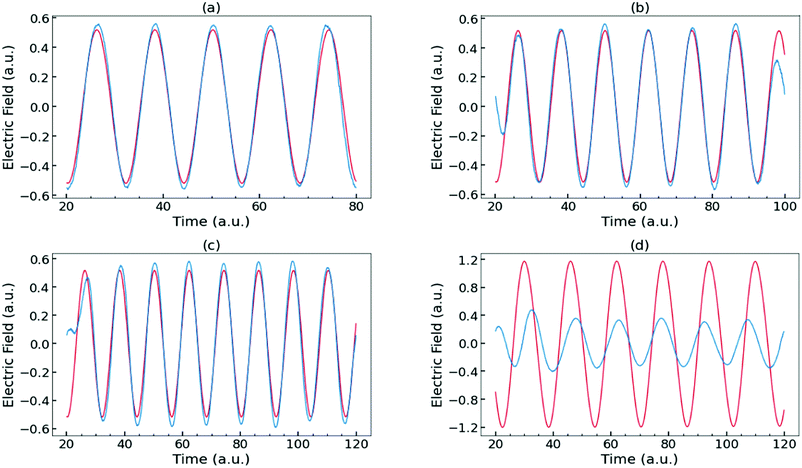Fig. 8 Comparisons of true (red) and machine-learned predicted (blue) E(t) fields. The electric fields correspond to the same potential, but with (a) 600, (b) 800, and (c) 1000 units. (d) True (red) and machine-learned (blue) E(t) for a different potential characterized by a large amplitude. When the true E(t) has a much larger amplitude, the machine learning algorithm is able to still accurately predict the resonance frequency but underestimates the amplitude.

To quantitatively demonstrate that the machine-learned and true E(t) are in excellent agreement, a fast Fourier transform was applied to both of these data sets. The amplitude and frequency of σ(ω) were then compared for each data point in the validation and test set. As before, we computed R2 values (cf.eqn (5)) for each of our 600-, 800-, and 1000-output-layer-unit models, and all of these configurations showed similar R2 statistics, which are summarized in Table 4. The loss and R2 of the training and validation sets were recorded every 1000 epochs. The figures in the ESI show that the 1000-output-layer-unit DNN was sufficiently trained at ∼50000 epochs, and further training introduces overfitting (∼30000 and ∼40000 epochs were required for the 600- and 800-output-layer-unit DNN to reach a minimal loss). It is also worth mentioning that batch normalization and dropout approaches (among others) are machine-learning techniques that could also be used to prevent overfitting of the data; however, since we did not observe any severe overfitting of our training set, we did not employ these techniques in our work. Nevertheless, the R2 for predicting the frequency on the validation set converged to an impressive ∼0.95 value for all three models (cf. ESI), and both Fig. 8 and 9 show that reducing the number of units in the layers of our cross-correlation neural network approach did not adversely affect its predictive performance.

Table 4 FNN metrics for predicting E(t) in the time domain with the 600-, 800-, and 1000-output-layer-unit models
Number of output layer units 600 800 1000
Number of epochs for best performance ∼30000 ∼40000 ∼50000
Loss on original test set 25.0653 44.5876 68.2800
R 2 for amplitude on pruned test set 0.3702 0.2594 0.1485
R 2 for frequency on original test set 0.9550 0.9381 0.9370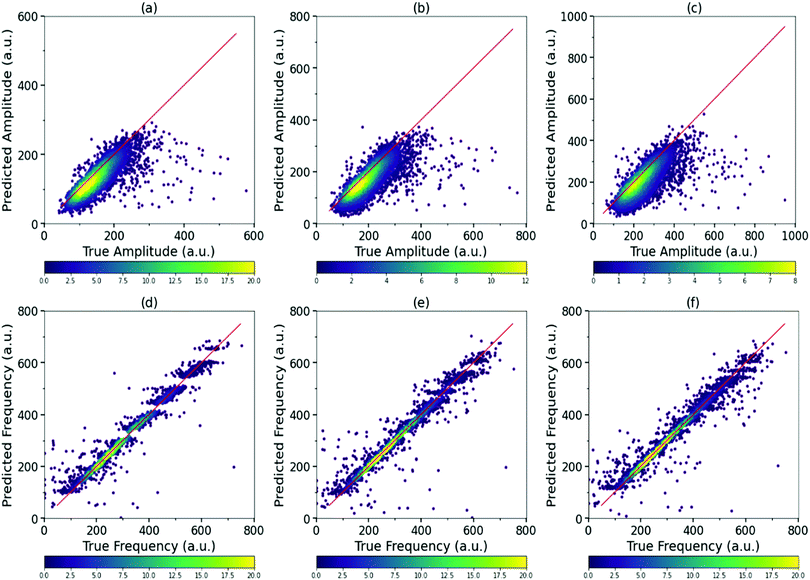Fig. 9 Scatter density plots of the predicted and true amplitude for the (a) 600-, (b) 800-, and (c) 1000-output-layer-unit model, respectively. Scatter density plots of the predicted and true resonance frequency for the (d) 600-, (e) 800-, and (f) 1000-output-layer-unit model, respectively. The diagonal line in each plot represents a perfect match between the machine-learned predictions and true values. The horizontal color bar in each sub-plot indicates the density of the data points.

In addition, we also investigated the effect of using only 2 hidden layers to predict E(t) with 1000 units. As shown in the ESI, the density plot obtained with a 2-hidden-layer FNN was more sparse and spread out. Furthermore, the R2 values for predicting the frequency on the validation set never exceeded 0.87, showing that the 2-hidden-layer neural network underfitted the data (cf. ESI). On the other hand, we also recognized that increasing the number of hidden layers beyond 3 would possibly improve the accuracy of our neural network; however, this modification would also incur an immense computational cost. Specifically, training our 3-hidden-layer FNN to predict E(t) required ∼256 GB of RAM and 20 hours on high-performance GPUs. Further training with additional layers would require even more memory and GPU time, which we felt was impractical since we already obtained impressive R2 values greater than 0.95 with our 3-hidden-layer FNN. As such, these benchmark configuration tests indicated that the use of 3 hidden layers in our neural network was sufficient and practical for accurate predictions. Most importantly, the density plots in Fig. 9 show that both the resonance frequencies and amplitudes predicted by our cross-correlation neural network approach demonstrate an impressive agreement with the brute-force (and computationally expensive) quantum control results obtained with the NIC-CAGE program.

## V. Conclusion

In conclusion, we have presented the first machine learning effort for solving explicit time-dependent quantum control problems in reduced-dimensional chemical systems. Using a variety of deep neural networks, we have shown that the prediction of optimal control fields is an inverse problem that naturally lends itself to a machine learning approach. In terms of efficiency, we have shown that our machine learning approach only requires knowledge of the potential, V(x), to yield a reliable prediction of an optimal control field, E(t). In other words, a user can simply input a variety of potentials into our neural network model to obtain optimal control fields without having to do a computationally expensive time-dependent quantum control calculation. In terms of accuracy, we have shown that deep neural networks can predict these optimal control fields within 96% accuracy by directly learning the underlying patterns between V(x) and E(t).

While this work focused on reduced-dimensional quantum systems, we anticipate that the machine learning techniques explored in this work could be applied to other applications of increasing complexity. For example, we envision that some of the machine learning tactics used here could serve as a first step towards solving more complex quantum dynamics problems in higher dimensions. The use of reduced-dimensional techniques to address full 3D quantum dynamics problems is similar in spirit to ongoing efforts that use machine-learned, ground-state, 1D exchange–correlation functionals42,45 for full three-dimensional chemical problems.46 Finally, we also anticipate that the machine learning techniques used here could be harnessed to predict optimal electric fields for other higher-lying transitions, which are known to exhibit more complex patterns in the time and frequency domains.41 In particular, cross-correlation neural network approaches, which were used to overcome problems associated with the random phase of E(t), could be useful in (1) predicting optimal electric fields for other higher-energy excitations in the time domain or (2) enabling the prediction of the full absorption/emission spectra of molecules since the absorption spectra is merely the Fourier transform of E(t). Taken together, these machine learning techniques show a promising path towards cost-effective statistical approaches for designing control fields that enable desired transitions in quantum dynamical systems.

## Conflicts of interest

There are no conflicts to declare.

## Acknowledgements

The quantum control calculations and generation of datasets in this work were supported by the National Science Foundation under Grant No. CBET-1833218. The machine learning calculations and algorithm developments in this work were supported by the National Science Foundation under Grant No. CHE-1808242. We gratefully acknowledge the support of NVIDIA Corporation with the donation of GPUs used for this research.

## References

1. G. M. Huang, T. J. Tarn and J. W. Clark, J. Math. Phys., 1983, 24, 2608–2618 CrossRef.
2. M. B. Oviedo and B. M. Wong, J. Chem. Theory Comput., 2016, 12, 1862–1871 CrossRef.
3. N. V. Ilawe, M. B. Oviedo and B. M. Wong, J. Chem. Theory Comput., 2017, 13, 3442–3454 Search PubMed.
4. N. V. Ilawe, M. B. Oviedo and B. M. Wong, J. Mater. Chem. C, 2018, 6, 5857–5864 RSC.
5. M. Maiuri, M. B. Oviedo, J. C. Dean, M. Bishop, B. Kudisch, Z. S. D. Toa, B. M. Wong, S. A. McGill and G. D. Scholes, J. Phys. Chem. Lett., 2018, 9, 5548–5554 Search PubMed.
6. B. Kudisch, M. Maiuri, L. Moretti, M. B. Oviedo, L. Wang, D. G. Oblinsky, R. K. Prud'homme, B. M. Wong, S. A. McGill and G. D. Scholes, Proc. Natl. Acad. Sci. U. S. A., 2020, 117, 11289–11298 CrossRef.
7. K. C. Nowack, F. H. L. Koppens, Y. V. Nazarov and L. M. K. Vandersypen, Science, 2007, 318, 1430–1433 Search PubMed.
8. M. Kues, C. Reimer, P. Roztocki, L. R. Cortés, S. Sciara, B. Wetzel, Y. Zhang, A. Cino, S. T. Chu, B. E. Little, D. J. Moss, L. Caspani, J. Azaña and R. Morandotti, Nature, 2017, 546, 622–626 CrossRef.
9. E. M. Fortunato, M. A. Pravia, N. Boulant, G. Teklemariam, T. F. Havel and D. G. Cory, J. Chem. Phys., 2002, 116, 7599–7606 CrossRef.
10. H. J. Williams, L. Caldwell, N. J. Fitch, S. Truppe, J. Rodewald, E. A. Hinds, B. E. Sauer and M. R. Tarbutt, Phys. Rev. Lett., 2018, 120, 163201 CrossRef.
11. A. Bartana, R. Kosloff and D. J. Tannor, Chem. Phys., 2001, 267, 195–207 CrossRef.
12. B. L. Brown, A. J. Dicks and I. A. Walmsley, Phys. Rev. Lett., 2006, 96, 173002 CrossRef.
13. M. J. Wright, J. A. Pechkis, J. L. Carini, S. Kallush, R. Kosloff and P. L. Gould, Phys. Rev. A: At., Mol., Opt. Phys., 2007, 75, 051401 Search PubMed.
14. P. Brumer and M. Shapiro, Acc. Chem. Res., 1989, 22, 407–413 Search PubMed.
15. J. Somlói, V. A. Kazakov and D. J. Tannor, Chem. Phys., 1993, 172, 85–98 Search PubMed.
16. W. Zhu, J. Botina and H. Rabitz, J. Chem. Phys., 1998, 108, 1953–1963 Search PubMed.
17. N. Khaneja, T. Reiss, C. Kehlet, T. Schulte-Herbrüggen and S. J. Glaser, J. Magn. Reson., 2005, 172, 296–305 CrossRef.
18. W. Zhu and H. Rabitz, J. Chem. Phys., 1998, 109, 385–391 CrossRef.
19. A. Raza, S. Bardhan, L. Xu, S. S. R. K. C. Yamijala, C. Lian, H. Kwon and B. M. Wong, Environ. Sci. Technol. Lett., 2019, 6, 624–629 CrossRef.
20. K. Gubaev, E. V. Podryabinkin and A. V. Shapeev, J. Chem. Phys., 2018, 148, 241727 CrossRef.
21. K. K. Yalamanchi, V. C. O. van Oudenhoven, F. Tutino, M. Monge-Palacios, A. Alshehri, X. Gao and S. M. Sarathy, J. Phys. Chem. A, 2019, 123, 8305–8313 Search PubMed.
22. K. Ryan, J. Lengyel and M. Shatruk, J. Am. Chem. Soc., 2018, 140, 10158–10168 Search PubMed.
23. B. Huang and O. A. von Lilienfeld, J. Chem. Phys., 2016, 145, 161102 Search PubMed.
24. Y. Liu, N. Marcella, J. Timoshenko, A. Halder, B. Yang, L. Kolipaka, M. J. Pellin, S. Seifert, S. Vajda, P. Liu and A. I. Frenkel, J. Chem. Phys., 2019, 151, 164201 CrossRef.
25. T. M. Dieb, Z. Hou and K. Tsuda, J. Chem. Phys., 2018, 148, 241716 CrossRef.
26. B. Himmetoglu, J. Chem. Phys., 2016, 145, 134101 CrossRef.
27. P. O. Dral, J. Phys. Chem. Lett., 2020, 11, 2336–2347 CAS.
28. J. Behler, J. Chem. Phys., 2016, 145, 170901 Search PubMed.
29. P. von den Hoff, S. Thallmair, M. Kowalewski, R. Siemering and R. de Vivie-Riedle, Phys. Chem. Chem. Phys., 2012, 14, 14460–14485 CAS.
30. S. Thallmair, D. Keefer, F. Rott and R. de Vivie-Riedle, J. Phys. B: At., Mol. Opt. Phys., 2017, 50, 082001 CrossRef.
31. T. Brixner and G. Gerber, ChemPhysChem, 2003, 4, 418–438 CrossRef CAS.
32. M. Dantus and V. V. Lozovoy, Chem. Rev., 2004, 104, 1813–1860 CAS.
33. B. M. Wong and S. Raman, J. Comput. Chem., 2007, 28, 759–766 CAS.
34. B. M. Wong, M. M. Fadri and S. Raman, J. Comput. Chem., 2008, 29, 481–487 CAS.
35. H. Bechtel, A. Steeves, B. Wong and R. Field, Angew. Chem., Int. Ed., 2008, 47, 2969–2972 CrossRef CAS.
36. B. M. Wong, Phys. Chem. Chem. Phys., 2008, 10, 5599–5606 RSC.
37. B. M. Wong, R. L. Thom and R. W. Field, J. Phys. Chem. A, 2006, 110, 7406–7413 CAS.
38. G. Reinisch, K. Miki, G. L. Vignoles, B. M. Wong and C. S. Simmons, J. Chem. Theory Comput., 2012, 8, 2713–2724 CAS.
39. B. M. Wong, A. H. Steeves and R. W. Field, J. Phys. Chem. B, 2006, 110, 18912–18920 CAS.
40. K. Prozument, R. G. Shaver, M. A. Ciuba, J. S. Muenter, G. B. Park, J. F. Stanton, H. Guo, B. M. Wong, D. S. Perry and R. W. Field, Faraday Discuss., 2013, 163, 33–57 RSC.
41. A. Raza, C. Hong, X. Wang, A. Kumar, C. R. Shelton and B. M. Wong, Comput. Phys. Commun., 2021, 258, 107541 CrossRef CAS.
42. L. Li, J. C. Snyder, I. M. Pelaschier, J. Huang, U.-N. Niranjan, P. Duncan, M. Rupp, K.-R. Müller and K. Burke, Int. J. Quantum Chem., 2016, 116, 819–833 CrossRef CAS.
43. M. Abadi, A. Agarwal, P. Barham, E. Brevdo, Z. Chen, C. Citro, G. S. Corrado, A. Davis, J. Dean, M. Devin, S. Ghemawat, I. Goodfellow, A. Harp, G. Irving, M. Isard, Y. Jia, R. Jozefowicz, L. Kaiser, M. Kudlur, J. Levenberg, D. Mané, R. Monga, S. Moore, D. Murray, C. Olah, M. Schuster, J. Shlens, B. Steiner, I. Sutskever, K. Talwar, P. Tucker, V. Vanhoucke, V. Vasudevan, F. Viégas, O. Vinyals, P. Warden, M. Wattenberg, M. Wicke, Y. Yu and X. Zheng, TensorFlow: Large-Scale Machine Learning on Heterogeneous Systems, 2015, http://tensorflow.org/, Software available from http://tensorflow.org Search PubMed.
44. N. Whitehead and A. Fit-Florea, Precision and performance: Floating point and IEEE 754 compliance for NVIDIA GPUs, 2019, https://docs.nvidia.com/cuda/index.html, CUDA libraries available from http://www.nvidia.com Search PubMed.
45. J. C. Snyder, M. Rupp, K. Hansen, K.-R. Müller and K. Burke, Phys. Rev. Lett., 2012, 108, 253002 CrossRef.
46. F. Brockherde, L. Vogt, L. Li, M. E. Tuckerman, K. Burke and K.-R. Müller, Nat. Commun., 2017, 8, 872 CrossRef.

### Footnotes

Electronic supplementary information (ESI) available. See DOI: 10.1039/d0cp03694c
X. W. and A. K. contributed equally to this work.
 This journal is © the Owner Societies 2020